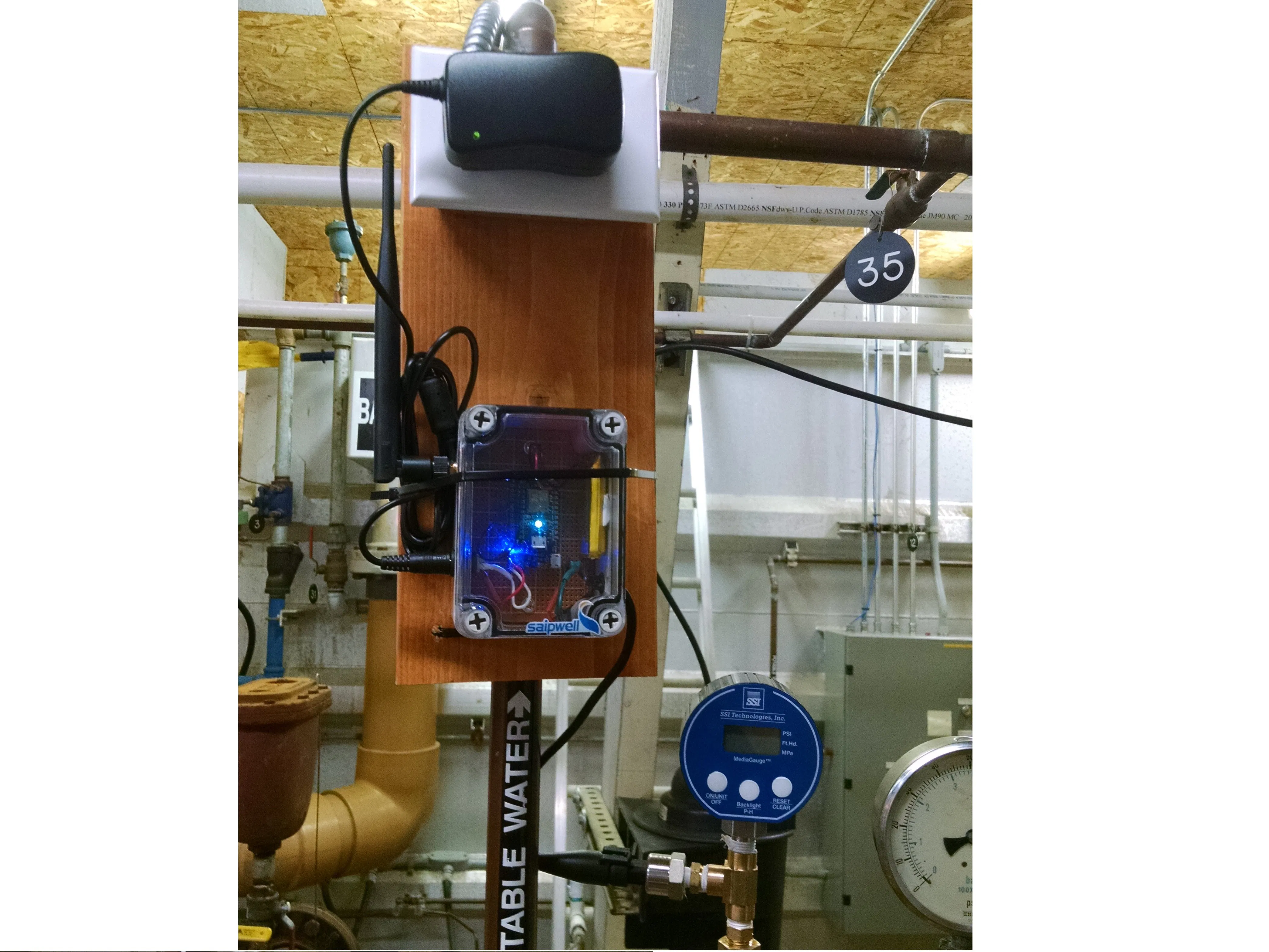# PSI Monitor for a Water Plant

A way to monitor the PSI of a water system remotely.

IntermediateShowcase (no instructions)5 hours982## Things used in this project

### Hardware componentsParticle Photon
×1
 Particle PhotonPower Shield
×1
 Project Box
×1
 Antenna and Pigtail
×1
 Autex Automotive Pressure Sensor
×1
 Power Connector (Generic)
×1
 Proto Board
×1
 12V Power Supply
×1

### Hand tools and fabrication machines

 Drill (Generic)
 Variable Bench Power Supply (Generic)
 Voltage Meter (Generic)Soldering iron (generic)
 Wire Cutters (Generic)

## Code

### psilinearregression.ino

C/C++
Code used to push psi, gallons in tank, and feet of water in tank to Ubidots and Particle cloud.
```#include <Ubidots.h>

#define TOKEN "[insert your token here]"

Ubidots ubidots(TOKEN);

//initialize variables
double a3avg;
double psiavg;
double psiavg30;
unsigned int countSec;
double bigPicture;

//start timer
Timer timer(5000, Update20TimesAMinute);

/*
Returns the PSI which can then be displayed,
or used to calculate other variables
@param The raw data
@return The PSI
*/
double Psi(double data)
{
return data*0.0304-12.313;
}

/*
Returns the number of gallons in the tank
which can then be displayed,
or used to calculate other variables
(new conversion psi to gal)
@param The PSI
@return The number of gallons in the tank
*/
return (12922.0*data)-878548.96;
}

/*
Returns the feet of water in the tank
which can then be displayed.
psi to ft (technically gal to ft)
@param The PSI
@return Feet of water in the tank
*/
{
}

/*
Returns a value fron data between
the desired min and max
@param input/data
@param minimum
@param maximum
@return clamped data
*/
double clamp(double input, double min, double max){
if(input>max){
return max;
}
if(input<min){
return min;
}
return input;
}

/*
Return the calculated a3avg.
@param raw data from a3
@return a3avg
*/
double updatea3avg(double a3){
return a3avg*0.95 + a3*0.05;
}

/*
Return the calculated psiavg.
over 5 seconds.
@param PSI
@return psiavg
*/
double updatePsiavg(double psi){
return psiavg*0.95 + psi*0.05;
}

/*
Return the calculated psiavg30.
over 1 minute 40 seconds.
@param PSI
@return psiavg
*/
double updatePsiavg30(double psi){
return psiavg30*0.998 + psi*0.002;
}

/*
Makes a more granular average by
only updating every minute.
Used for zooming out on ubidots
without the page freezing.
*/
void updateBigPicture(){
if(countSec%12==0){
bigPicture=psiavg30;
}

}

/*
Pushes variables to Particle.io
*/
void updateParticle(){
Particle.variable("psi", &psiavg, DOUBLE);
Particle.variable("psiavg30", &psiavg30, DOUBLE);

}

/*
Pushes variables to Ubidots
*/
void updateUbidots(double psi){

ubidots.sendAll();

}

/*
Sets up program to run:
Enables the external antenna
Sets up time for counting
Sets variables to useful numbers
Starts timer
Pushes data to particle
*/
void setup() {
STARTUP(WiFi.selectAntenna(ANT_EXTERNAL));
Particle.syncTime();

a3avg = (double) analogRead(A3);
psiavg = Psi(a3avg);
psiavg30 = psiavg;

adjGal= AdjPSIToGallons(psiavg30);//number of gallons in the tank
adjFt=AdjPSIToFt(psiavg30); //hight of the water in the tank

countSec=0;
updateBigPicture();

timer.start();

updateParticle();
}
/*
Vestigial. Required but not used.
*/
void loop() {

}

/*
Main method.
Gets raw data and uses it to update
and push variables.
*/
void Update20TimesAMinute()
{
int a3 = analogRead(A3);
double psi = Psi(a3);

a3avg = updatea3avg(a3);
psiavg = updatePsiavg(psi);
psiavg30 =updatePsiavg30(psi);

updateBigPicture();
updateUbidots(psi);

}
```

## Credits

### Katelyn Rule

1 project • 3 followers